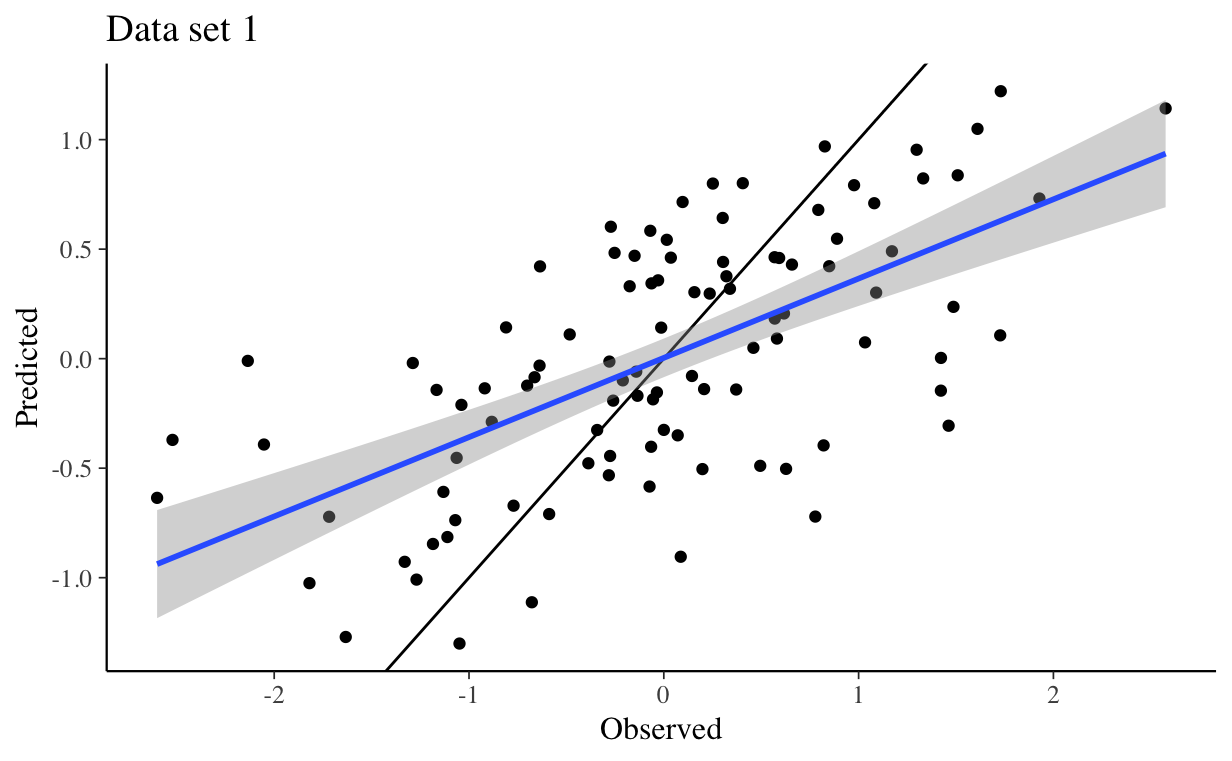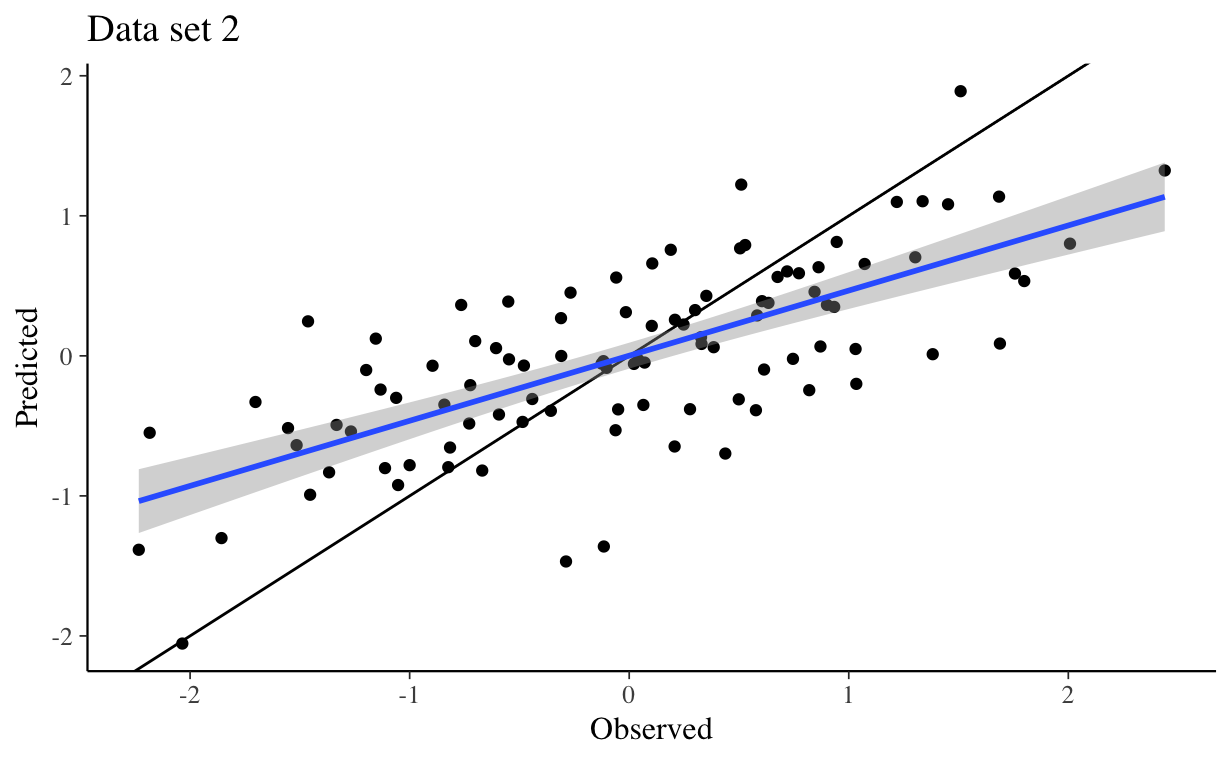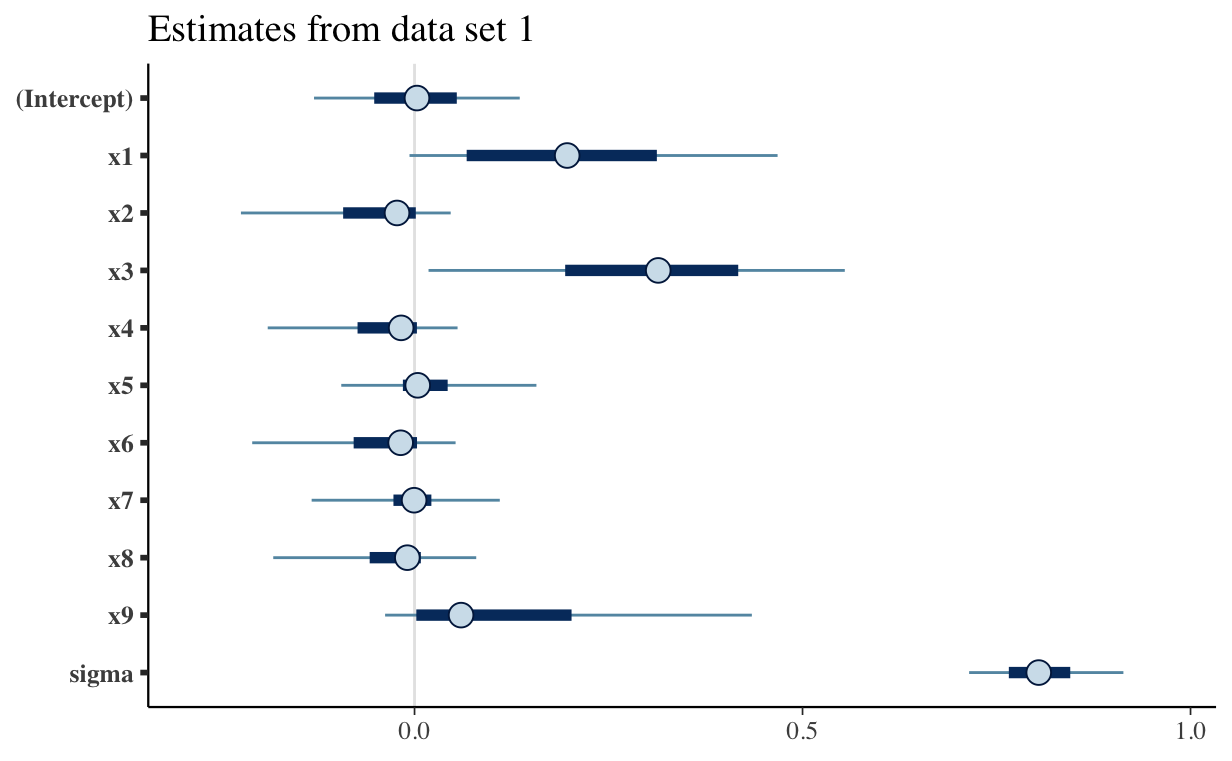It won’t come as a surprise to anyone who knows me that I have to try a Bayesian approach to variable selection. It also turns out that there’s an easy way to do it, since “horseshoe priors” are built into rstanarm. What’s a horeshoe prior you ask? Well you can see a few of the details in the next section, or you can just skip to the section where we use it in rstanarm if you find the math more confusing than helpful.

## What is a horsehoe prior?

If you really want to know and understand horseshoe priors, you’ll need to read a paper by Juho Pironen and Aki Vehtari in the Electronic Journal of Statistics,1 but here’s a brief outline.

The likelihood in standard linear regression model looks like this

\eqalign{ y_i &\sim& \mbox{N}(\mu_i, \sigma^2) \\ \mu_i &=& \beta_0 + \sum_j \beta_jx_{ij} }

To complete the Bayesian model, we need to specify priors for $$\sigma^2$$ and the $$\beta$$s. The default choices in rstanarm are:2

\eqalign{ \sigma &\sim& \mbox{Exp}(1) \\ \beta_0 &\sim& \mbox{N}(0, 10) \\ \beta_i &\sim& \mbox{N}(0, 2.5) \quad \mbox{for } i > 0 }

These are the choices we used here. The basic horsehoe prior affects only the last of these. Specifically,

\eqalign{ \beta_i &\sim& \mbox{N}(0, \tau^2\lambda_i^2) \\ \lambda_i &\sim& \mbox{Cauchy}^+(0, 1) \quad , }

where $$\mbox{Cauchy}^+$$ refers to a half-Cauchy distribution on the positive real line. A Cauchy distribution has very fat tails, so the tendency for any $$\beta_i$$ is to be either close to zero, because $$\lambda_i$$ is small, or well away from zero, because $$\lambda_i$$ is large. $$\tau$$ sets the total amount of influence the covariates have on the response, rather like $$\lambda$$ in the Lasso.

As Pironen and Vehtari explain, however, there hasn’t been consensus on how to set or estimate $$\tau$$. They introduce a new version of the horseshoe prior, the regularized horseshoe prior that looks like this

\eqalign{ \beta_i &\sim& \mbox{N}(0, \tau^2\tilde\lambda_i^2) \\ \tilde\lambda_i &=& \frac{c^2\lambda_i^2}{c^2 + \tau^2\lambda_i^2} \\ \lambda_i &\sim& \mbox{Cauchy}^+(0, 1) \quad . }

Notice that if $$\tau^2\lambda_i^2 \ll c^2$$, meaning that $$\beta_i$$ is close to 0, then we have something very close to the original horseshoe prior. If on the other hand, $$\tau^2\lambda_i^2 \gg c^2$$ then the prior on $$\beta_i$$ is close to $$\mbox{N}(0, c^2)$$. Now we just3 need a prior on $$c$$. Read Pironen and Vehtari if you want all of the gory details. We’ll just use hs() as a prior in our model, setting the global_scale parameter according to their advice.

## Setting up the data

Once again we have to start by generating the data.

library(tidyverse)
library(reshape2)
library(ggplot2)
library(cowplot)
library(mvtnorm)
library(corrplot)

rm(list = ls())
## intetcept
##
beta0 <- 1.0
## regression coefficients
##
beta <- c(1.0, -1.0, 1.0, 0.0, 0.0, 0.0, 0.0, 0.0, 0.0)
## pattern of correlation matrix, all non-zero entries are set to saem
## correlation, covariance matrix caldulated from individual variances and a
## single association parameter governing the non-zero correlation coefficients
##
## Note: Not just any pattern will work here. The correlation matrix and
## covariance matrix generated from this pattern must be positive definite.
## If you change this pattern, you may get an error when you try to generate
## data with a non-zero association parameter.
##
Rho <- matrix(nrow = 9, ncol = , byrow = TRUE,
data = c(1,0,1,0,1,0,1,0,1,
0,1,0,1,0,1,0,1,0,
1,0,1,0,1,0,1,0,1,
0,1,0,1,0,1,0,1,0,
1,0,1,0,1,0,1,0,1,
0,1,0,1,0,1,0,1,0,
1,0,1,0,1,0,1,0,1,
0,1,0,1,0,1,0,1,0,
1,0,1,0,1,0,1,0,1
))
## vector of standard deviations for covariates
##
sigma <- rep(1, 9)

## construct a covariance matrix from the pattern, standard deviations, and
## one parameter in [-1,1] that governs the magnitude of non-zero correlation
## coefficients
##
## Rho - the pattern of associations
## sigma - the vector of standard deviations
## rho - the association parameter
##
construct_Sigma <- function(Rho, sigma, rho) {
## get the correlation matris
##
Rho <- Rho*rho
for (i in 1:ncol(Rho)) {
Rho[i,i] <- 1.0
}
## notice the use of matrix multiplication
##
Sigma <- diag(sigma) %*% Rho %*% diag(sigma)
return(Sigma)
}

## set the random number seed manually so that every run of the code will
## produce the same numbers
##
set.seed(1234)

n_samp <- 100
cov_str <- rmvnorm(n_samp,
mean = rep(0, nrow(Rho)),
sigma = construct_Sigma(Rho, sigma, 0.8))

resid <- rep(2.0, n_samp)

y_str <- rnorm(nrow(cov_str), mean = beta0 + cov_str %*% beta, sd = resid)
dat_1 <- data.frame(y_str, cov_str, rep("Strong", length(y_str)))

cov_str <- rmvnorm(n_samp,
mean = rep(0, nrow(Rho)),
sigma = construct_Sigma(Rho, sigma, 0.8))
y_str <- rnorm(nrow(cov_str), mean = beta0 + cov_str %*% beta, sd = resid)
dat_2 <- data.frame(y_str, cov_str, rep("Strong", length(y_str)))

column_names <- c("y", paste("x", seq(1, length(beta)), sep = ""), "Scenario")
colnames(dat_1) <- column_names
colnames(dat_2) <- column_names

## saving results in scale allows me to use them later for prediction with
## new data
##
scale_1 <- lapply(dat_1[, 1:10], scale)
scale_2 <- lapply(dat_2[, 1:10], scale)

## when assigning the same scaling to a data frame, the scaling attributes
## are lost
##
dat_1[, 1:10] <- lapply(dat_1[, 1:10], scale)
dat_2[, 1:10] <- lapply(dat_2[, 1:10], scale)

## Trying the horseshoe prior

Now that we have the data, let’s try the horshoe prior.

library(rstanarm)

options(mc.cores = parallel::detectCores())

set.seed(1234)

## n is the number of observations
## D is the number of covariates
## p0 is the expected number of important covariates
##
n <- nrow(dat_1)
D <- ncol(dat_1[,2:10])
p0 <- 3
tau0 <- p0/(D - p0) * 1/sqrt(n)
prior_coeff <- hs(global_scale = tau0, slab_scale = 1)

fit_1 <- stan_glm(y ~ x1 + x2 + x3 + x4 + x5 + x6 + x7 + x8 + x9,
data = dat_1,
prior = prior_coeff,
refresh = 0,
## Note: I use the same prior scale here because both data sets have the same number of observations
## and the same expected number of important covariates
##
fit_2 <- stan_glm(y ~ x1 + x2 + x3 + x4 + x5 + x6 + x7 + x8 + x9,
data = dat_2,
prior = prior_coeff,
refresh = 0,

predict_1 <- posterior_predict(fit_1)
predict_2 <- posterior_predict(fit_2)

for.plot <- data.frame(Observed = dat_1$y, Predicted = apply(predict_1, 2, mean)) p <- ggplot(for.plot, aes(x = Observed, y = Predicted)) + geom_point() + geom_abline(slope = 1, intercept = 0) + geom_smooth(method = "lm") + ggtitle("Data set 1") print(p)for.plot <- data.frame(Observed = dat_2$y, Predicted = apply(predict_2, 2, mean))
p <- ggplot(for.plot, aes(x = Observed, y = Predicted)) +
geom_point() +
geom_abline(slope = 1, intercept = 0) +
geom_smooth(method = "lm") +
ggtitle("Data set 2")
print(p)cat("Results from data set 1\n")
Results from data set 1
summary(fit_1, digits = 3)

Model Info:

function:     stan_glm
family:       gaussian [identity]
formula:      y ~ x1 + x2 + x3 + x4 + x5 + x6 + x7 + x8 + x9
algorithm:    sampling
priors:       see help('prior_summary')
sample:       4000 (posterior sample size)
observations: 100
predictors:   10

Estimates:
mean     sd       2.5%     25%      50%      75%      97.5%
(Intercept)      0.002    0.081   -0.159   -0.052    0.003    0.054    0.166
x1               0.202    0.153   -0.022    0.067    0.197    0.312    0.508
x2              -0.051    0.086   -0.267   -0.092   -0.023    0.002    0.073
x3               0.303    0.159    0.000    0.194    0.314    0.417    0.595
x4              -0.039    0.078   -0.234   -0.073   -0.017    0.003    0.086
x5               0.017    0.077   -0.131   -0.015    0.004    0.043    0.204
x6              -0.043    0.081   -0.248   -0.078   -0.018    0.003    0.083
x7              -0.006    0.075   -0.187   -0.027   -0.001    0.022    0.148
x8              -0.027    0.079   -0.223   -0.058   -0.009    0.008    0.119
x9               0.117    0.155   -0.066    0.002    0.060    0.202    0.514
sigma            0.808    0.060    0.700    0.766    0.804    0.845    0.937
mean_PPD         0.000    0.113   -0.229   -0.075    0.001    0.075    0.219
log-posterior -177.435    4.780 -187.876 -180.399 -177.095 -174.074 -169.109

Diagnostics:
mcse  Rhat  n_eff
(Intercept)   0.001 1.000 5339
x1            0.003 1.000 2125
x2            0.002 1.000 2634
x3            0.003 1.000 2334
x4            0.001 1.000 3196
x5            0.001 1.000 4265
x6            0.001 1.000 2960
x7            0.001 0.999 4140
x8            0.001 1.000 3831
x9            0.004 1.001 1807
sigma         0.001 1.000 3766
mean_PPD      0.002 1.000 4337
log-posterior 0.137 1.001 1211

For each parameter, mcse is Monte Carlo standard error, n_eff is a crude measure of effective sample size, and Rhat is the potential scale reduction factor on split chains (at convergence Rhat=1).
cat("\n\nResults from data set 2\n")


Results from data set 2
summary(fit_2, digits = 3)

Model Info:

function:     stan_glm
family:       gaussian [identity]
formula:      y ~ x1 + x2 + x3 + x4 + x5 + x6 + x7 + x8 + x9
algorithm:    sampling
priors:       see help('prior_summary')
sample:       4000 (posterior sample size)
observations: 100
predictors:   10

Estimates:
mean     sd       2.5%     25%      50%      75%      97.5%
(Intercept)      0.000    0.074   -0.145   -0.050    0.001    0.050    0.147
x1               0.118    0.120   -0.037    0.016    0.091    0.198    0.392
x2              -0.172    0.164   -0.511   -0.298   -0.142   -0.022    0.046
x3               0.467    0.135    0.186    0.386    0.474    0.560    0.709
x4               0.005    0.078   -0.163   -0.025    0.001    0.033    0.180
x5               0.018    0.073   -0.119   -0.014    0.005    0.045    0.197
x6              -0.259    0.172   -0.570   -0.390   -0.272   -0.116    0.019
x7               0.009    0.070   -0.131   -0.018    0.002    0.035    0.171
x8               0.010    0.076   -0.139   -0.019    0.002    0.037    0.192
x9              -0.011    0.072   -0.190   -0.035   -0.002    0.019    0.131
sigma            0.740    0.056    0.641    0.700    0.735    0.777    0.860
mean_PPD         0.000    0.108   -0.211   -0.073   -0.001    0.072    0.214
log-posterior -169.089    4.782 -179.092 -172.021 -168.731 -165.702 -160.714

Diagnostics:
mcse  Rhat  n_eff
(Intercept)   0.001 1.000 4646
x1            0.002 1.000 2371
x2            0.004 1.002 1576
x3            0.002 0.999 3194
x4            0.001 0.999 4414
x5            0.001 1.000 4767
x6            0.004 1.002 1531
x7            0.001 1.000 4473
x8            0.001 0.999 4565
x9            0.001 1.000 4384
sigma         0.001 0.999 3974
mean_PPD      0.002 1.000 4471
log-posterior 0.141 1.002 1158

For each parameter, mcse is Monte Carlo standard error, n_eff is a crude measure of effective sample size, and Rhat is the potential scale reduction factor on split chains (at convergence Rhat=1).

There are several things I like about using regularized horeshoe priors in rstanarm rather than the Lasso.

1. I get an assessment of how reliable estimates of the regression coefficients are in addition to a point estimate of what they are. In this case you can see that the 95% credible intervals overlap 0 for every coefficient in analysis of the first data set, while only the credible intervals for x3 don’t overlap 0 in analysis of the second data set. Notice, however, that some of the intervals are very close to not overlapping 0, i.e., the intervals for x1, x3, and x9 in the analysis of data set 1 and the intervals for x1, x2, and x6 in the analysis of data set 2.

2. I can also plot the estimates and their uncertainty very easily. Plotting the estimates and their uncertainty makes is much easier to pick out the covariates that seem to have an association with the response variable.4 Note: The outer intervals in these plots correspond to 90% credible intervals, not 95% credible intervals. You could change that by specifying prob_outer = 0.95 in the call to plot().

p <- plot(fit_1) + ggtitle("Estimates from data set 1")
print(p)p <- plot(fit_2) + ggtitle("Estimates from data set 2")
print(p)